# Soal Matematika Mtk 1. Let Be “it Is Cold” And

1. Let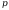be It is cold and let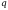be It is raining

Give a simple verbal sentence which describes each of the following statements:

 a.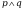b.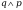c.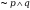d.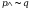e.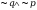f.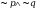g.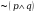h.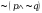i.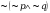2. Letbe Marc is rich and letbe Marc is happy. Write each of the following in symbolic form.

 a. Marc is rich and happy. b. Marc is rich but happy. c. Marc is poor but happy d. Marc is rich but unhappy e. It is not true that Marc rich and happy f. It is not true that Marc poor but unhappy

1. Find the value of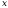so that each of the following statements is true,

a.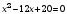and 5 + 21 = 26

b.factor of 12 and sin 120 =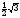c.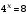and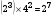d.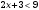and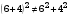e.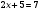and 25 is a square number

f. sin=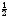and 7 is a prime number

g.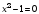and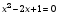h.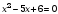and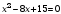3. Letbe He is tall and letbe He is handsome.

Write each of the following statements in symbolic form usingand.

a. He is tall but not handsome. (.)

b. It is false that he is short or handsome. (.)

c. He is neither tall nor handsome. (.)

d. He is tall, or he is short and handsome. (.)

e. It is true that he is short or not handsome. (.)